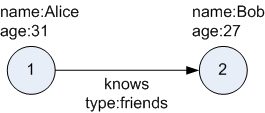## 1.1.1 What Are Property Graphs?

A property graph consists of a set of objects or vertices, and a set of arrows or edges connecting the objects. Vertices and edges can have multiple properties, which are represented as key-value pairs.

Each vertex has a unique identifier and can have:

• A set of outgoing edges

• A set of incoming edges

• A collection of properties

Each edge has a unique identifier and can have:

• An outgoing vertex

• An incoming vertex

• A text label that describes the relationship between the two vertices

• A collection of properties

For vertices and edges, each property is identified with a unique name.

The following figure illustrates a very simple property graph with two vertices and one edge. The two vertices have identifiers 1 and 2. Both vertices have properties `name` and `age`. The edge is from the outgoing vertex 1 to the incoming vertex 2. The edge has a text label `knows` and a property `type` identifying the type of relationship between vertices 1 and 2.

Figure 1-1 Simple Property Graph ExampleDescription of "Figure 1-1 Simple Property Graph Example"

A property graph can have self-edges (that is, an edge whose source and destination vertex are the same), as well as multiple edges between the same source and destination vertices.

A property graph can also have different types of vertices and edges in the same graph. For example a graph can have a set of vertices with label `Person` and a set of vertices with label `Place`, with different properties relevant to these two sets of vertices.

The property graph data model is similar to the W3C standards-based Resource Description Framework (RDF) graph data model; however, the property graph data model is simpler and less precise than RDF.

The property graph data model features and analytic APIs make property graphs a good candidate for use cases such as these:

• Identifying influencers in a social network

• Predicting trends and customer behavior

• Discovering relationships based on pattern matching

• Identifying clusters to customize campaigns

Note:

The property graph data model that Oracle supports at the database side does not allow labels for vertices. However, you can treat the value of a designated vertex property as one or more labels.

Related Topics